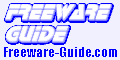Home & EducationMathematicsJMathLib
JMathLib is used to evaluate complex mathematical expressions and display the results graphically. It can be used either interactively or to interpret script files (m-files). It is a clone of Matlab, Octave, FreeMat, Scilab but written 100% in java.
More...

Isoptikon
The application is devoted to the precise drawing (on screen and printer) of figures of Euclidean Geometry and the study of the so called 'isoptic' cubics.
More...

ZGrapher
ZGrapher 1.4 (last freeware version) is an awards winning graphing and calculating software.
More...

PEEI
The program PEEI calculates a discrete, numerical and approximate solution of a generic differential analytical model (i.e. a system of partial differential equations).
More...

MathCast
MathCast is an equation editor, an application that allows you to input mathematical equations. These equations can be used in written documents, webpages, and even databases. They could be rendered graphically to the screen, to picture files, or to MathML - todays leading standard language for describing mathematics. MathCast can be used freely by anyone: students can create equation sheets to help them in their studies, educators can write handouts or study guides, webmasters can add mathematics to their website, and the list goes on and on.

Graph Explorer
A free graph plotting program. Enter a symbolic expression and see the corresponding polar or cartesian graph. Zoom-in, zoom-out and paste the graphs into other documents.

SimSolve
A fast, general purpose and free simultaneous equation solver. Just specify the number of variables, enter the coefficients, and press solve. Reports the numerical solution if only one exists, describes the constraints if infinite solutions exist, and tells you it's impossible if no solution exists. The 'show workings' mode explains how the program came to its conclusions.

FreeMat
FreeMat is a free environment for rapid engineering and scientific prototyping and data processing. It is similar to commercial systems such as MATLAB from Mathworks, and IDL from Research Systems, but is Open Source. FreeMat is available under the GPL license.

Maxima
Maxima is a system for the manipulation of symbolic and numerical expressions, including differentiation, integration, Taylor series, Laplace transforms, ordinary differential equations, systems of linear equations, polynomials, and sets, lists, vectors, matrices, and tensors. Maxima yields high precision numeric results by using exact fractions, arbitrary precision integers, and arbitrarily precision floating point numbers. Maxima can plot functions and data in two and three dimensions.

GraphCalc
GraphCalc 1.21 (last freeware version) is an all-in-one solution to everything from everyday arithmetic to statistical analysis, from betas to Booleans, from cubes to calculus, from decimals to derivatives. GraphCalc combines all the features of a professional mathematics package with the simplicity of an easy to learn windows interface. It provides user-friendly help and tutorials to guide you through the easy and fun process of mastering GraphCalc.

SpeQ
SpeQ is a small, extensive mathematics program with a simple, intuitive interface. All calculations are entered in a sheet. In there you can freely add, edit and execute all calculations. You can define variables and functions, and plot graphs of your functions. You can save your calculations for later re-use.
Features:
- Expression calculations with arithmetic, conditional, logical and bitwise operators
- Around 100 built-in mathematical, physical and conditional constants and colors
- Around 60 built-in functions for Analysis, Arithmetic, Complex, Hyperbolic, Integers, Probability, Statistics, Trigonometry
- Define your own variables and functions for use in calculations
- Plot fully adaptable graphs, trace the plotted functions, save your graphs
- Complex number calculations
- Colored workarea
- Orderly overview of all available functions and constants in the Functionstree
- A list with all defined variables and functions in the Memorylist
- Context-sensitive help and a detailed users guide.Home & Education
Calculators
Calendars & Planners
Chemistry
Food & Beverage
Genealogy
Geography
Health & Nutrition
Hobbies
Home Inventory
Kids & Parenting
Language
Mathematics
Miscellaneous
Religion & Spirituality
Science
Teaching Tools